# Example of Assignment: Cycles with Conditional Statement

Calculate the value of the function y, representing function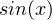in Taylor series. The argumentvaries from -2 to 2 in increments of 0.5. Identify the error.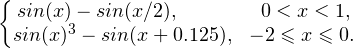Block scheme of the solution: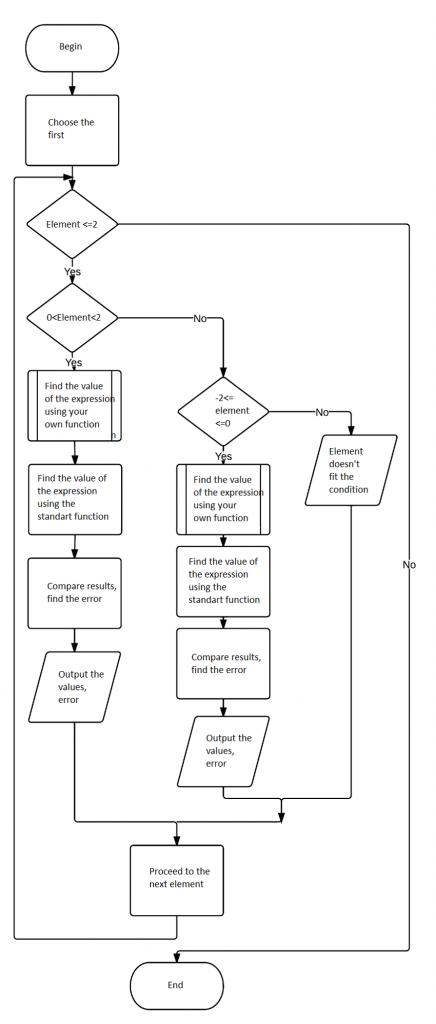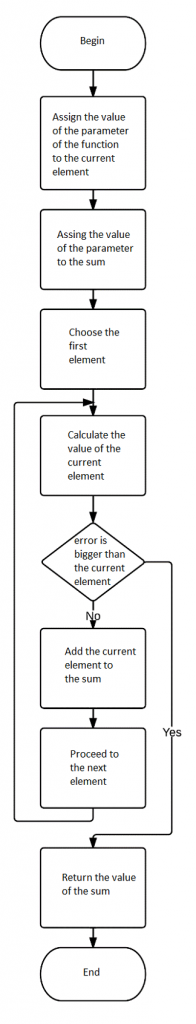C++ implementation:

#include<iostream>
#include<math.h>
#include<iomanip>
using namespace std;
double error;
double mysin(double x)//my sin function
{
double item = x, sum = x;
for (int i = 3;; i += 2)//endless cycle
{
item *= -1 * (x*x) / ((i - 1)*i);//Taylor series
if (fabs(item) <= error) break;//exit condition
sum += item;
}
return sum;
}
void main()
{
cout << "if 0<x<1 y=sinx-sin(x/2)\nif -2<=x<=0 y=sinx^3-sin(x+0.125)\n";
cout << "enter error="; cin >> error;//input error
double y, y2, error;
const double min = -2, max = 2, step = 0.5;
cout << setw(10) << "x" << setw(20) << "my sin" << setw(20) << "st. sin" << setw(20) << "error" << endl << endl;//output string-header
for (double x = min; x <= max; x += step)//cycle: x from -2 to 2 with step 0.5 { if (x>0 && x<1)
{
y = mysin(x) - mysin(x / 2);//my sin calculating
y2 = sin(x) - sin(x / 2);//standart sin calculating
error = fabs(y - y2);//compareing/calculating error
cout << setw(10) << x << setw(20) << y << setw(20) << y2 << setw(20) << error << endl;//output result } else if (x >= -2 && x <= 0)
{
y = pow(mysin(x), 3) - mysin(x + 0.125);//my sin calculating
y2 = pow(sin(x), 3) - sin(x + 0.125);//standart sin calculating
error = fabs(y - y2);//compareing/calculating error
cout << setw(10) << x << setw(20) << y << setw(20) << y2 << setw(20) << error << endl;//output result
}
else//if x doen't fit the condition
cout << setw(10) << x << setw(20) << "undefined" << setw(20) << "undefined" << endl;
}
system("pause");//pause console
}


Screenshot of the working program: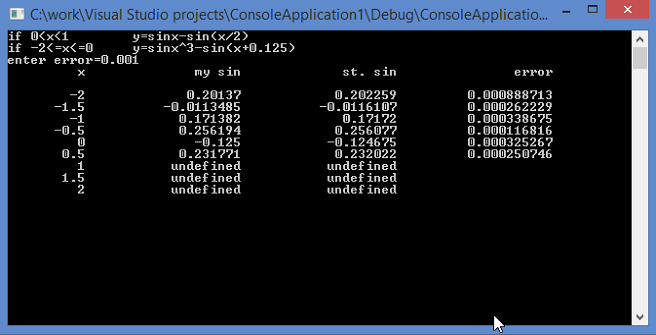This example of assignment includes information that can be helpful for dealing with your own assignment. Some students try to present our samples as their own assignments, but don’t do that, as you will be accused in plagiarism. If you like our example of assignment, you can get your homework done by AssignmentShark.com. Look at our page with testimonials to see that our customers remain satisfied with our service. Our experts help students with different disciplines. An assignment of any difficulty can be done by our professionals. Use our service any time you want, as we work 24/7. Get our help right now as we are ready to help you!

You can also like assignment example on cyclic functions.